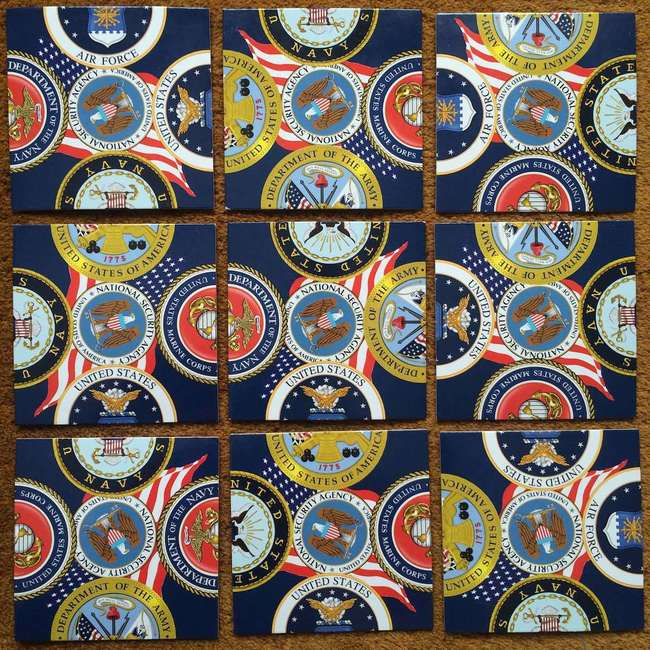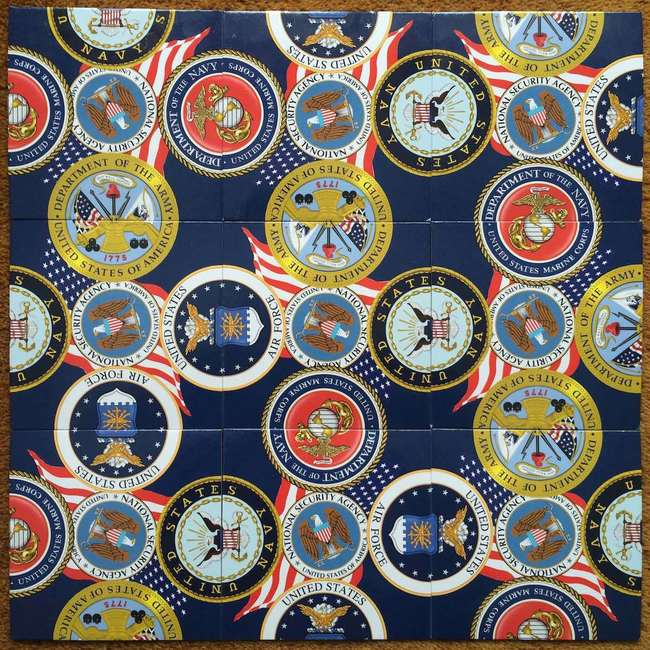# NSA puzzle

My dad recently presented me with a puzzle. The puzzle consists of nine square tiles. Each tile edge has the top or bottom half of an Air Force, Army, Marines, or Navy emblem. The challenge is to arrange the tiles in a 3x3 grid where all emblems between touching tiles align correctly.## Number of possibilities #

To create a 3x3 grid, we start by choosing a tile for the upper-left hand corner. We have 9 tiles to choose from. Each one of those tiles can also be rotated in one of 4 ways (0, 90, 180 and 270 degrees). Therefore we have 9 * 4 = 36 options for the first tile. Next we choose the upper-middle tile. With the upper-left tile selected, we only have 8 tiles to choose from. Once again, this tile can be rotated one of 4 ways. Therefore we have 8 * 4 = 32 options for the second tile. Proceeding like this we have 7 * 4 = 28 options for the upper-right tile, 6 * 4 = 24 options for the middle-left tile and so on. We can compute the total number of possibilities to be more than 95 billion!

``````= (9*4)*(8*4)*(7*4)*(6*4)*(5*4)*(4*4)*(3*4)*(2*4)*(1*4)
= 9*8*7*6*5*4*3*2*1*4*4*4*4*4*4*4*4*4
= 9! * 4^9
= 95,126,814,720*
``````

*Actually the number of possibilities is one fourth the 95 billion. Imagine you arrange one of the possible solutions on the floor. Now rotate this entire 3x3 grid 90, 180 or 270 degrees. All the locations where edges touch are still the same. Therefore our 95 billion arrangements assemble each unique 3x3 solution four times. The number of possibilities is actually 23,781,703,680. Regardless, if we could assemble one of these 23+ billion arrangements per second, it would take over 750 years to try them all.

## Data structure #

Computers can perform billions of operations per second. They are perfectly suited for trying the tile arrangements. However, laptop computers can’t see emblems or move tiles. They work well with numbers. First we must represent the tiles numerically. The system of values I devised is this:

``````Air Force emblem    top half: 1, bottom half: -1
Army emblem         top half: 2, bottom half: -2
Marines emblem      top half: 3, bottom half: -3
Navy emblem         top half: 4, bottom half: -4
``````

Each tile has four edges. Going clockwise from the top let us refer to these edges as north, east, south and west. We represent each tile as a four item list. Each item in the list corresponds to an edge `[north, east, south, west]`. Here’s one tile example:

``````= [Air Force top, Navy bottom, Marines bottom, Army top]
= [1, -4, -3, 2]
``````

Rotating this tile clockwise is achieved by shifting each value to the right and wrapping around

``````[ 1, -4, -3,  2]  # original
[ 2,  1, -4, -3]  # rotated 90 degrees
[-3,  2,  1, -4]  # rotated 180 degrees
[-4, -3,  2,  1]  # rotated 270 degrees
``````

To check if tiles align, we check that the values along touching edges are opposite (-4 and 4 for example). We can also add the values and they should equal zero.

## Implementation #

I used Python to code the solution. The tiles are represented as a list of deque’s. A deque is a data structure for doing fast rotation of list elements. We will need to rotate the tiles frequently so the deque is a good choice. The nine tiles in the puzzle are as follows:

``````tiles = [
deque([ 2,-2,-4,-3]),
deque([-3,-4, 3, 2]),
deque([-2, 1,-1,-4]),
deque([-1, 1,-4, 3]),
deque([-3, 1,-4,-2]),
deque([ 3, 4, 2, 1]),
deque([-2,-3, 1, 4]),
deque([ 2,-4,-3, 1]),
deque([-3,-1, 2, 4])
]
``````

Python has a great permutation library, so in order to calculate all possible tile placement positions, we just permutate our list of tiles:

``````perms = list(permutations(tiles))
``````

At this point a naive approach may be to take one tile arrangement and check if all edges align. If they do not, try a different tile arrangement. Keep trying until all 95 billion arrangements are checked. Four of them will work (remember the aligned 3x3 grid can be rotated 4 different ways)

We can save a lot of time by placing the tiles one by one instead. For any given tile ordering, first check if the upper-middle tile’s left (west) edge aligns with the upper-left tile’s right (east) edge. If it does not, there’s no need to keep checking if the remaining seven tiles align, including all their possible rotated positions. This saves us from checking seven additional tiles with four rotations each or 47 = 16,384 possibilities. Most placements will fail in the first few tiles saving us from exploring millions of possibilities.

We proceed by rotating the upper-middle tile clockwise until an edge matches the upper-left tile. If no edge matches, rotate the upper-left tile once and try rotating the upper-middle tile again checking for a match. If there’s a match, move on to the upper-right tile and on from there. If no matches exist for a single tile ordering, move on to the next tile ordering and begin rotating all over again. In Python:

``````for perm in perms:
for t1 in range(0, 4): # rotate
perm.rotate()
for t2 in range(0, 4):
perm.rotate()
if (perm + perm): # check edge
continue; # if edge mismatch don't check remaining tiles
for t3 in range(0, 4):
perm.rotate()
if (perm + perm):
continue;
for t4 in range(0, 4):
perm.rotate()
if (perm + perm):
continue;
.
.
.
for t9 in range(0, 4):
perm.rotate()
if ((perm + perm) |
(perm + perm)):
continue;
print "SOLVED!"
print perm
exit()
``````

Full source available here

## Solution #